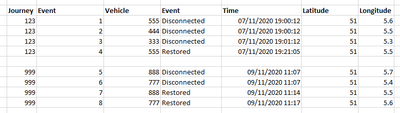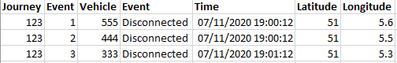cancel
Showing results for
Did you mean:Frequent Visitor

## Calculating the maximum distance across multiple rows?

Hello,

I have a bit of a complicated question about calculating distances across rows. I wanted to see if you can help out. First, I will explain the data and goal then go over an example of what I'm trying to do.

Sample dataJourney Event Vehicle Event Time Latitude Longitude 123 1 555 Disconnected 07/11/2020 19:00:12 51 5.6 123 2 444 Disconnected 07/11/2020 19:00:12 51 5.5 123 3 333 Disconnected 07/11/2020 19:01:12 51 5.3 123 4 555 Restored 07/11/2020 19:21:05 51 5.5 999 5 888 Disconnected 09/11/2020 11:07:03 51 5.7 999 6 777 Disconnected 09/11/2020 11:07:50 51 5.4 999 7 888 Restored 09/11/2020 11:14:36 51 5.5 999 8 777 Restored 09/11/2020 11:17:26 51 5.6

We have multiple journeys which contain multiple events that occur on different vehicles. Each event has a time it was created as well as coordinates of where it was created.

Goal

What I need to do: whenever there is a "Disconnected" event, I need to know what the distance of that vehicle is with the other vehicles and show the maximum of those distances.

Important

• There can be 1 or more vehicles per journey
• The "disconnected" event can occur more than once per journey per vehicle (so for example, once in the morning, maybe again an hour later).
• The math for distance between coordinates is not difficult, but the formula is long so I will simplify how I represent it in the below example by using DISTANCE[lat1, long1, lat2, long2].
• Additionally, as I think if you can sum or take the difference of values across the rows, you can probably calculate the distance as well, so the important thing to answer here is not "what formula gives me distances?" it's "how do I apply these formulas across the rows?"

Example with sample data• If we look at the first sample journey, 123, we see there are 3 vehicles (555, 444, 333).
• A "Disconnected" event has occured around 19:00 (events 1, 2, 3).
• For event 1 (row 1) I would like to know the maximum of the distances that vehicle has from the others:
• Event 1 vs Event 2 distance would be DISTANCE[51, 5.6, 51, 5.5]. Let's assume it's 50 km
• Event 1 vs Event 3 distance would be DISTANCE[51, 5.6, 51, 5.3]. Let's assume it's 60 km
• That means on the row for event 1 I would like to have a column with the value of 60.
• Note: important to remember that there could be another "Disconnected" event later on, for example 30 minutes later, where we would want to repeat this same calculation

Does anyone have any ideas?

1 ACCEPTED SOLUTIONCommunity Support

Hi  @sem1 ,

According to your description, create the data and set the formula rule to [latitude] + [longitude].1. Create Measure.

``````Measure =
var _table=GENERATE(SELECTCOLUMNS(FILTER(ALL('Table'),[Journey]=MAX([Journey])),"jo1",[Journey],"Type",[Vehicle],"juli",[Longitude]+[Latitude]),SELECTCOLUMNS(FILTER(ALL('Table'),[Journey]=MAX([Journey])),"jo2",'Table'[Journey],"Type2",[Vehicle],"juli2",[Longitude]+[Latitude]))

var _value=
MAXX(_table,ABS([juli]-[juli2]))

return
IF(MAX('Table'[Event.1])="Disconnected",_value,BLANK())``````

2. Result.Best Regards,

Liu Yang

If this post helps, then please consider Accept it as the solution to help the other members find it more quickly.Community Support

Hi  @sem1 ,

According to your description, create the data and set the formula rule to [latitude] + [longitude].1. Create Measure.

``````Measure =
var _table=GENERATE(SELECTCOLUMNS(FILTER(ALL('Table'),[Journey]=MAX([Journey])),"jo1",[Journey],"Type",[Vehicle],"juli",[Longitude]+[Latitude]),SELECTCOLUMNS(FILTER(ALL('Table'),[Journey]=MAX([Journey])),"jo2",'Table'[Journey],"Type2",[Vehicle],"juli2",[Longitude]+[Latitude]))

var _value=
MAXX(_table,ABS([juli]-[juli2]))

return
IF(MAX('Table'[Event.1])="Disconnected",_value,BLANK())``````

2. Result.Best Regards,

Liu Yang

If this post helps, then please consider Accept it as the solution to help the other members find it more quickly.